Courses

# Test: Analysis of Stress & Strain - 3

## 10 Questions MCQ Test Strength of Materials (SOM) | Test: Analysis of Stress & Strain - 3

Description
This mock test of Test: Analysis of Stress & Strain - 3 for Mechanical Engineering helps you for every Mechanical Engineering entrance exam. This contains 10 Multiple Choice Questions for Mechanical Engineering Test: Analysis of Stress & Strain - 3 (mcq) to study with solutions a complete question bank. The solved questions answers in this Test: Analysis of Stress & Strain - 3 quiz give you a good mix of easy questions and tough questions. Mechanical Engineering students definitely take this Test: Analysis of Stress & Strain - 3 exercise for a better result in the exam. You can find other Test: Analysis of Stress & Strain - 3 extra questions, long questions & short questions for Mechanical Engineering on EduRev as well by searching above.
QUESTION: 1

### A solid circular shaft is subjected to bending moment M and twisting moment T. What is the equivalent twisting moment Te which will produce the same maximum shear stress as the above combination?

Solution:

Maximum shear stress,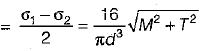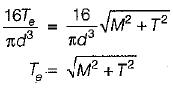QUESTION: 2

Solution:
QUESTION: 3

### The principal stresses at a point in an elastic material are 60 N/mm2 tensile, 20 N/mm2 tensile and 50 N/mm2 compressive. If the material properties are: μ = 0.35 and E = 105 N/mm2 then the volumetric strain of the material is

Solution:

Volumetric strain,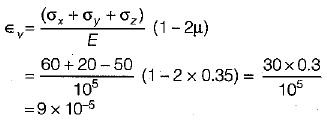QUESTION: 4

The state of stress at a point in a body under plane state of stress condition is given by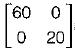MPa . The maximum shear stress (in MPa) is

Solution: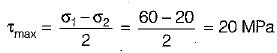QUESTION: 5

If at a point in a body σ= 70 MPa, σ= 60 MPa and τxy = - 5 MPa, then the radius of Mohr’s circle is equal to

Solution: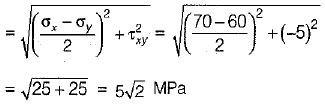QUESTION: 6

The state of stress at a point in a loaded member is shown in figure below. The magnitude of maximum shear stress is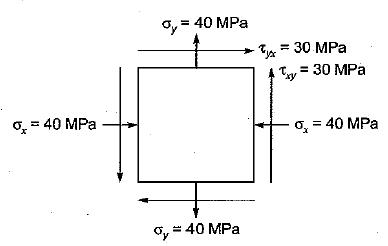Solution:

Maximum shear stress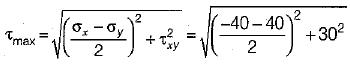= 50 MPa

QUESTION: 7

The cross-section of a bar is subjected to a uniaxial tensile stress 'p' The tangential stress on a plane inclined at θ to the cross-section of the bar would be

Solution:

Normal stress = pcos2θ
Tangential stress = psinθ cosθ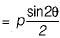QUESTION: 8

The figure shows the state of stress at a certain point in a stressed body. The magnitudes of normal stresses in the x and y directions are 100 MPa and 20 MPa respectively. The radius of Mohr’s stress circle representing this state of stress is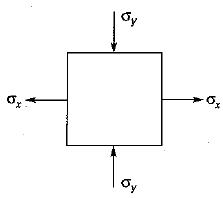Solution: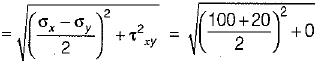= 60 MPa

QUESTION: 9

Match List-I (State of stress) with List-II (Kind of loading) and select the correct answer using the codes given below the lists:
List-I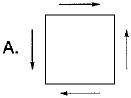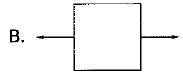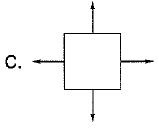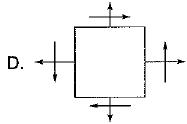List-II
1. Combined bending and torsion of circular shaft
2. Torsion of circular shaft
3. Thin cylinder subjected to internal pressure
4. The bar subjected to tensile force
Codes:
A B C D
(a) 2 1 3 4
(b) 3 4 2 1
(c) 2 4 3 1
(d) 3 1 2 4

Solution:

Torsion of circular shaft (pure shear)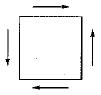Bar subjected to tensile force (shear will not be present)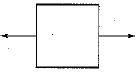Combined bending and torsion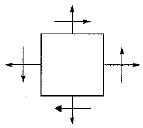Thin cylinder subjected to internal pressure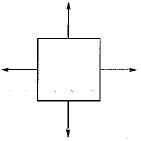QUESTION: 10

Consider the Mohr’s circle shown below. What is the state of stress represented by this circle?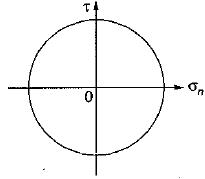Solution:

The state of stress is represented by σx = σy = 0, τxy ≠ 0